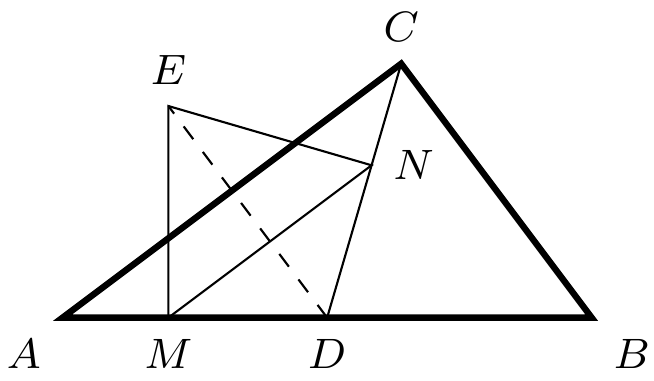TrigInTriangle Triangle PtolemyTheorem Circle Intermediate

Problem - 3140
As shown, $\angle{ACB} = 90^\circ$, $AD=DB$, $DE=DC$, $EM\perp AB$, and $EN\perp CD$. Prove $$MN\cdot AB = AC\cdot CB$$The solution for this problem is available for $0.99. You can also purchase a pass for all available solutions for$99.